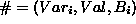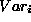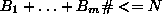Next: The range Library Up: The fd (Finite Domain) Previous: The fd Integer Arithmetic

### The fd Complex Constraints

There are two motivations for supporting complex constraints. One is to simplify problem modelling. It is shorter, and more natural, to use a single alldistinct constraint on N variables than to use n*(n-1)/2 (pairwise) disequalities!

The second motivation is to achieve specialised constraint propagation behaviour. The `alldistinct` constraint on N variables, has the same semantics as n*(n-1)/2 (pairwise) disequalities, but it can also achieve better propagation than would be possible with the disequalities. For example if any M of the variables have the same domain, and its size is less than M, then the `alldistinct` constraint can immediately fail. However if two variables X and Y have the same domain, with M>1 elements, the constraint X `##` Y can achieve no propagation at all. Thus the pairwise disequalities are unable to achieve the same propagation as the alldistinct constraint.

The constraint atmost(Number, List, Val) constrains atmost Number of the variables in the list List to take the value Val. This is a difficult constraint to express using logic. One way is to constrain each sublist of length Number+1 to contain a variable with value different from Val, but the resulting number of constraints can be very large!

A more natural way is to constrain all the variables to take a value different from Val, and to allow the constraint to be violated up to N times. The fd library supports such a facility with the constraint `#=`(T1, T2, B). This constraint makes B=1 if T1=T2 and B=0 otherwise. It is possible to express atmost by imposing the constraintfor each variablein the list and then adding the constraint. The built-in atmost constraint is essentially implemented like this.

The other fd constraints (`#<`, `#>`, etc.) can be extended with an extra 0/1 variable in the same way.

The fd library includes a great variety of facilities, which are best explored by obtaining the ECLiPSe extensions manual [Be97] and looking at the programming examples in the section on the fd library there.Next: The range Library Up: The fd (Finite Domain) Previous: The fd Integer Arithmetic

Joachim Schimpf
Wed Sep 3 18:07:19 BST 1997